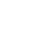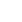# Roll the Dice – Probability & Statistics

JRDAssist
Middle School and High School Teacher

Students will learn about probability and statistics while rolling dice and flipping a coin carefully recording their results.

##Length of Time: Two 45 Minute Periods

### Common Core Alignment

CCSS.Math.Content.6.SP.B.5.A - Reporting the number of observations.

CCSS.Math.Content.6.SP.B.5.B - Describing the nature of the attribute under investigation, including how it was measured and its units of measurement.

CCSS.Math.Content.6.SP.B.5.C - Giving quantitative measures of center (median and/or mean) and variability (interquartile range and/or mean absolute deviation), as well as describing any overall pattern and any striking deviations from the overall pattern with reference to the context in which the data were gathered.

CCSS.Math.Content.6.SP.B.5.D - Relating the choice of measures of center and variability to the shape of the data distribution and the context in which the data were gathered.

## Objectives & Outcomes

The learners will be able to understand the concept of probability and use their results to make predictions in real world situations and applications.

## Materials Needed

• One die and coin for each student
• plain white paper
• pencil

## Procedure

### Opening to Lesson

• Give one die to each student in the classroom.
• Have them stand in a line at an empty table, desk, or floor area.
• Each one will roll the die, and depending on the number rolled they will be sent to a predetermined area of the room.  (Example:  roll 1, 2, 3 go to…, roll 4, 5, 6 go to…)  The students should be close to evenly divided.
• The teacher will ask a variety of questions to peak students’ interest such as, “Why did some of you roll sixes, and others roll ones?”  And other similar questions.
• Teacher will direct students back to their seats

### Body of Lesson

#### Modeling

• Teacher will demonstrate coin tossing and the chances of getting a heads or a tails.
• Teacher will display large copy of results chart and demonstrate how to record die information and coin tossing results

#### Guided Practice

• Each student will use their materials and flip the coin 100 times recording the results
• Student will then analyze the results and make a prediction for the next 100 flips
• Student will again record the results of the 100 flips
• Each student will use their materials and roll the die 100 times recording the results
• Student will then analyze the results and make a prediction for the next 100 rolls
• Student will again record the results of the 100 rolls
• The teacher will demonstrate how to show probability in fraction form

#### Independent Practice

• The teacher will give each student a worksheet illustrating a full deck of playing cards
• On a separate page there will be questions about the probability of choosing aces, diamonds, face cards, etc.
• The teacher will collect the completed page.  This will be used as an assessment.

### Closing

Ask students to give real world applications and situations of probability.  Prompt students for answers such as weather predictions, sports, and many others.

## Assessment & Evaluation

Using an illustrated handout (worksheet) with a set number of playing cards (each suit represented, face cards, etc.), the students will answer questions related to the probability of choosing certain named card, suits, etc.

## Modification & Differentiation

Students may work in pairs.  Use spinners instead of dice.  Introduce colored M&Ms or Skittles, or other examples of randomness.  Students could make graphs showing results.  Discuss variables that may change typical results.

## Related Lesson Plans

##### The Value of a Number

The students will work in groups of 4-6 physically learning and reviewing place value.

##### Comparing Multiplication Facts (Hey Tocayo!)

Students will be assigned a number that has various factors and they will find partners with different factors that have the same product.

##### Numbers By The Book

This lesson will allow students to show a relationship between numbers and a visual representation in a manner that can be used for younger students.

##### Fractions, Decimals, Percents, & Graphs

This lesson is designed to explore fractions, decimals, percent’s, and graphs with TRIX cereal.

##